## Sudoku Symmetry Group (minimal spec)

Everything about Sudoku that doesn't fit in one of the other sections

### Re: Proofiness

Mathimagics wrote:As for my rotation-based SG generator, it's failure on just N=4, N=6 is really intriguing.

BTW: if fails for N=2 too, with the "fix" to my code.
It's obvious why in that case ... 'x' is "do nothing" and so you'ld only get the rotations.
blue

Posts: 894
Joined: 11 March 2013

### Re: Sudoku Symmetry Group (minimal spec)

.
Re: Proofiness!

Ok, I see, now that is seriously cool.

Nice job, blue ...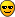PS: it seems that my rotate version does have some practical advantages! It seems to simplify the Schreier-Sims algorithm in some sort of way. So GAP can verify N=12 for the mine, but not for yours.

Now I have to see if I can get to 16 (this is very unlikely, but it's nice to know what the actual limit is.)

Update: It blew up on N = 13. Tragic!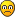Mathimagics
2017 Supporter

Posts: 1533
Joined: 27 May 2015
Location: Canberra

### Re: Sudoku Symmetry Group (minimal spec)

You can get farther, probably faster too, if you use wreath products instead of cell permutations.
The cells method deals with permutations of N^4 objects, but using wreath products it's only 2*N^2.
If you need cell permutations at some point, there's a way to convert from one representation to the other.
Checking N=2,...,16 takes ~20 minutes using wreath products.
If you have questions, they'ld have to wait until after Christmas.

Merry Christmas (to all),
Blue.

P.S. Last night, I started checks for both methods in "for N in [2..100]" loops.
In the morning, ~6h in, they were both working on N=21, with 5-600M of RAM in use ... ~10% more for my generators.

P.P.S. Other news: MinimalGeneratingSet() reports that for N=3 and N=4, SudokuP also needs only 3 generators !
SmallGeneratingSet() reports the same for N=5,..20.
blue

Posts: 894
Joined: 11 March 2013

### Re: Sudoku Symmetry Group (minimal spec)

blue wrote:The cells method deals with permutations of N^4 objects, but using wreath products it's only 2*N^2.

If you added a tail to that idea it would surely be a fox!That is seriously cunning. You could run a book on what value of N will finally make GAP blow a gasket ... perhaps near N = 112?

blue wrote: ... for N=3 and N=4, SudokuP also needs only 3 generators ! SmallGeneratingSet() reports the same for N=5,..20.

Cool, I'll try some generator constructions while you're off wassailing ... I'm a Hare Krishna (Branch Rastafararian), we don't do XmasCheers!Mathimagics
2017 Supporter

Posts: 1533
Joined: 27 May 2015
Location: Canberra

### Re: Sudoku Symmetry Group (minimal spec)

.
Ok, a simple modification to my Sudoku Symmetry Group generator works for SudokuP.

Sudoku SG generator:

• x = ShiftR(N-2) + ShiftB(N-1)
• y = ShiftR(N-1) + ShiftB(N-2)
• z = Rotate

First I changed the ShiftR operations to ShiftRB - this denotes applying the same row shift in all bands. Thus ShiftRB(K) = cyclic shift the first K rows of all bands.

Then I simply added ReflectMR, which denotes the mini-row "transposition" applied in each band, aka "transformation F". This can be applied to either x or y (if both are F-ed the group size halves).

Thus we get:
SudokuP SG generator:

• x = ShiftRB(N-2) + ShiftB(N-1) + ReflectMR
• y = ShiftRB(N-1) + ShiftB(N-2)
• z = Rotate

This has been verified for N = 3 to 15.

N = 16 crashes GAP. (Can we still use WreathProduct's here?)Mathimagics
2017 Supporter

Posts: 1533
Joined: 27 May 2015
Location: Canberra

### Re: Sudoku Symmetry Group (minimal spec)

Nice work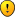Can we still use WreathProduct's here?

Yes. The permutations act on 4*N points instead of N^4.

WreathProduct(SymmetricGroup(N), P) works ... with a few options for the permutation group, P.

P = Group((1,3)(2,4), (1,4))
P = Group((1,4)(2,3), (1,2))

For irregular box sizes ... (Nr x Nc), you can use
DirectProduct(WreathProduct(SymmetricGroup(Nr), SymmetricGroup(2)), WreathProduct(SymmetricGroup(Nc), SymmetricGroup(2)));

With Nr=Nc=N, that would correspond to P = Group((1,2), (3,4)) ... with ReflectMR and ReflectMC, but no Rotate and no Flip.
blue

Posts: 894
Joined: 11 March 2013

### Re: Sudoku Symmetry Group (minimal spec)

.
Thanks blue.

How is that "N in [2..200]" iteration progressing?

What does the individual iteration time growth look like? Can it actually finish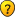Mathimagics
2017 Supporter

Posts: 1533
Joined: 27 May 2015
Location: Canberra

### Re: Sudoku Symmetry Group (minimal spec)

N in [2..89] took ~1 hour.
[2..200] might finish, but probably not for days.

The times aren't very reproducible -- maybe due to "randomized" methods being used (?).
Here are two runs for N in [5,10..50].
Hidden Text: Show
Code: Select all
`gap> pCheck(PG2, [5,10..50]);true for PG2 : N=5 (0 ms)true for PG2 : N=10 (0 ms)true for PG2 : N=15 (31 ms)true for PG2 : N=20 (94 ms)true for PG2 : N=25 (265 ms)true for PG2 : N=30 (1922 ms)true for PG2 : N=35 (4172 ms)true for PG2 : N=40 (5188 ms)true for PG2 : N=45 (8796 ms)true for PG2 : N=50 (20422 ms)gap> pCheck(PG2, [5,10..50]);true for PG2 : N=5 (0 ms)true for PG2 : N=10 (0 ms)true for PG2 : N=15 (47 ms)true for PG2 : N=20 (78 ms)true for PG2 : N=25 (282 ms)true for PG2 : N=30 (1828 ms)true for PG2 : N=35 (3687 ms)true for PG2 : N=40 (4641 ms)true for PG2 : N=45 (8531 ms)true for PG2 : N=50 (15750 ms)gap>`
blue

Posts: 894
Joined: 11 March 2013

### Re: Sudoku Symmetry Group (minimal spec)

.
The Schreier-Sims algorithm implementation in GAP definitely involves some randomising (there is a Wikipedia page on this).

Of course, for those of us who are running on Windows, ALL runtimes are non-reproducible ...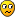Mathimagics
2017 Supporter

Posts: 1533
Joined: 27 May 2015
Location: Canberra

### Re: Sudoku Symmetry Group (minimal spec)

Mathimagics wrote:The Schreier-Sims algorithm implementation in GAP definitely involves some randomising (there is a Wikipedia page on this).

I knew that from the GAP docs.
Garbage collection was my other idea, since I did the two runs "back to back", instead of after each after a "clean" startup.

Of course, for those of us who are running on Windows, ALL runtimes are non-repr[oducible ...Yeah... A 15-vs-20 second difference wouldn't be due to Windows, though.

--

Other news: For irregular box sizes, (Nr x Nc), the Sudoku and SudokuP groups need 4 generators (assuming Nr and Nc are >= 2).

For Sudoku, these work:

• w = ShiftR(Nr) + ShiftS(Nr-1)
• x = ShiftC(Nc) + ShiftB(Nc-1)
• y = ShiftR(Nr-1) + ShiftS(Nr)
• z = ShiftC(Nc-1) + ShiftB(Nc)
For SudokuP, these work: (with ShiftR == your ShiftRB, and similar for ShiftC)

• w = ShiftR(Nr) + ShiftS(Nr-1)
• x = ShiftC(Nc) + ShiftB(Nc-1)
• y = ReflectMR
• z = ReflectMC

Added: related to the above, these work for SudokuP with square boxes:

• x = ShiftR(N) + ShiftS(N-1)
• y = ReflectMR
• z = Flip
blue

Posts: 894
Joined: 11 March 2013

### Re: Sudoku Symmetry Group (minimal spec)

blue wrote:For irregular box sizes, (Nr x Nc), the Sudoku and SudokuP groups need 4 generators (assuming Nr and Nc are >= 2).

Sudoku and SudokuP generators for rectangular boxes, that 's cool!The need for an extra generator is to be expected, no?Mathimagics
2017 Supporter

Posts: 1533
Joined: 27 May 2015
Location: Canberra

### Re: Sudoku Symmetry Group (minimal spec)

Mathimagics wrote:The need for an extra generator is to be expected, no?

I'm not sure how to answer that.
For normal Suduku, intuitively, the answer is "yes", I suppose.

I probably said more than I should have, when I used the word "needs".
GAP's MinimalGeneratingSet() reports that it's true for Nr,Nc in {2,3,4}, for both types.
If one of the number is >= 5, it gives the message about the group not being solvable, and suggests using SmallGeneratingSet().

For SudokuP / "Disjoint Groups", like it was with 9x9, "ReflectMR * ReflectMC" can be accomplished with a "Flip" followed by row and column permutations. The intermediate steps don't involve valid grids though. Still, the fact that a transpose is involved, hints that maybe somehow, in some cases, 3 generators could work (?).
blue

Posts: 894
Joined: 11 March 2013

Previous